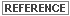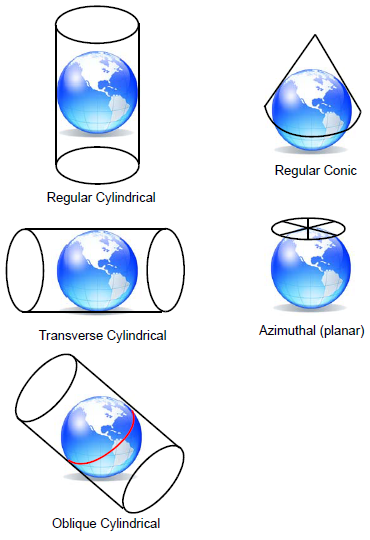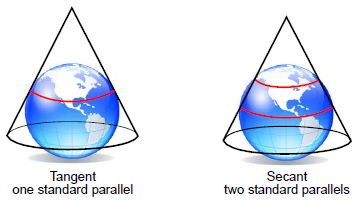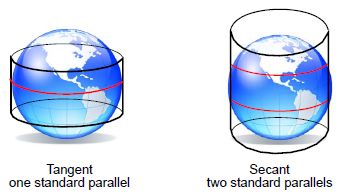# Projections

## Producer Field Guide

HGD_Product
Producer Field Guide
HGD_Portfolio_Suite
Producer

A map projection is the manner in which the spherical surface of the Earth is represented on a flat (two-dimensional) surface. This can be accomplished by direct geometric projection or by a mathematically derived transformation. There are many kinds of projections, but all involve transfer of the distinctive global patterns of parallels of latitude and meridians of longitude onto an easily flattened surface, or developable surface.

The three most common developable surfaces are the cylinder, cone, and plane. See the "Projection Types" figure below. A plane is already flat, while a cylinder or cone may be cut and laid out flat, without stretching. Thus, map projections may be classified into three general families: cylindrical, conical, and azimuthal or planar.This section is adapted from "Map Projections for Use with the Geographic Information System" by Lee and Walsh (Lee and Walsh, 1984).

Map projections are selected in the Projection Chooser. For more information about the Projection Chooser, see ERDAS IMAGINE Help.

Properties of Map Projections

Regardless of what type of projection is used, it is inevitable that some error or distortion occurs in transforming a spherical surface into a flat surface. Ideally, a distortion-free map has four valuable properties:

• conformality
• equivalence
• equidistance
• true direction

Each of these properties is explained below. No map projection can be true in all of these properties. Therefore, each projection is devised to be true in selected properties, or most often, a compromise among selected properties. Projections that compromise in this manner are known as compromise projections.

Conformality is the characteristic of true shape, wherein a projection preserves the shape of any small geographical area. This is accomplished by exact transformation of angles around points. One necessary condition is the perpendicular intersection of grid lines as on the globe. The property of conformality is important in maps which are used for analyzing, guiding, or recording motion, as in navigation. A conformal map or projection is one that has the property of true shape.

Equivalence is the characteristic of equal area, meaning that areas on one portion of a map are in scale with areas in any other portion. Preservation of equivalence involves inexact transformation of angles around points and thus, is mutually exclusive with conformality except along one or two selected lines. The property of equivalence is important in maps that are used for comparing density and distribution data, as in populations.

Equidistance is the characteristic of true distance measuring. The scale of distance is constant over the entire map. This property can be fulfilled on any given map from one, or at most two, points in any direction or along certain lines. Equidistance is important in maps that are used for analyzing measurements (that is, road distances). Typically, reference lines such as the equator or a meridian are chosen to have equidistance and are termed standard parallels or standard meridians.

True direction is characterized by a direction line between two points that crosses reference lines (for example, meridians) at a constant angle or azimuth. An azimuth is an angle measured clockwise from a meridian, going north to east. The line of constant or equal direction is termed a rhumb line.

The property of constant direction makes it comparatively easy to chart a navigational course. However, on a spherical surface, the shortest surface distance between two points is not a rhumb line, but a great circle, being an arc of a circle whose center is the center of the Earth. Along a great circle, azimuths constantly change (unless the great circle is the equator or a meridian).

Thus, a more desirable property than true direction may be where great circles are represented by straight lines. This characteristic is most important in aviation. Note that all meridians are great circles, but the only parallel that is a great circle is the equator.

Projection TypesProjection Types

Although a great number of projections have been devised, the majority of them are geometric or mathematical variants of the basic direct geometric projection families described below. Choice of the projection to be used depends upon the true property or combination of properties desired for effective cartographic analysis.

Azimuthal Projections

Azimuthal projections, also called planar projections, are accomplished by drawing lines from a given perspective point through the globe onto a tangent plane. This is conceptually equivalent to tracing a shadow of a figure cast by a light source. A tangent plane intersects the global surface at only one point and is perpendicular to a line passing through the center of the sphere. Thus, these projections are symmetrical around a chosen center or central meridian. Choice of the projection center determines the aspect, or orientation, of the projection surface.

Azimuthal projections may be centered:

• on the poles (polar aspect)
• at a point on the equator (equatorial aspect)
• at any other orientation (oblique aspect)

The origin of the projection lines—that is, the perspective point—may also assume various positions. For example, it may be:

• the center of the Earth (gnomonic)
• an infinite distance away (orthographic)
• on the Earth’s surface, opposite the projection plane (stereographic)

Conical Projections

Conical projections are accomplished by intersecting, or touching, a cone with the global surface and mathematically projecting lines onto this developable surface.

A tangent cone intersects the global surface to form a circle. Along this line of intersection, the map is error-free and possess equidistance. Usually, this line is a parallel, termed the standard parallel.

Cones may also be secant, and intersect the global surface, forming two circles that possess equidistance. In this case, the cone slices underneath the global surface, between the standard parallels. Note that the use of the word secant, in this instance, is only conceptual and not geometrically accurate. Conceptually, the conical aspect may be polar, equatorial, or oblique. Only polar conical projections are supported in ERDAS IMAGINE.

Tangent and Secant ConesCylindrical Projections

Cylindrical projections are accomplished by intersecting, or touching, a cylinder with the global surface. The surface is mathematically projected onto the cylinder, which is then cut and unrolled.

A tangent cylinder intersects the global surface on only one line to form a circle, as with a tangent cone. This central line of the projection is commonly the equator and possesses equidistance.

If the cylinder is rotated 90 degrees from the vertical (that is, the long axis becomes horizontal), then the aspect becomes transverse, wherein the central line of the projection becomes a chosen standard meridian as opposed to a standard parallel. A secant cylinder, one slightly less in diameter than the globe, has two lines possessing equidistance.

Tangent and Secant CylindersPerhaps the most famous cylindrical projection is the Mercator, which became the standard navigational map. Mercator possesses true direction and conformality.

Other Projections

The projections discussed so far are projections that are created by projecting from a sphere (the Earth) onto a plane, cone, or cylinder. Many other projections cannot be created so easily.

Modified projections are modified versions of another projection. For example, the Space Oblique Mercator projection is a modification of the Mercator projection. These modifications are made to reduce distortion, often by including additional standard lines or a different pattern of distortion.

Pseudo projections have only some of the characteristics of another class projection. For example, the Sinusoidal is called a pseudocylindrical projection because all lines of latitude are straight and parallel, and all meridians are equally spaced. However, it cannot truly be a cylindrical projection, because all meridians except the central meridian are curved. This results in the Earth appearing oval instead of rectangular (Environmental Systems Research Institute, 1991).